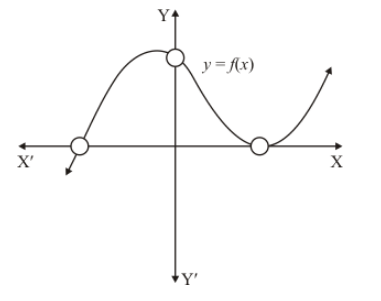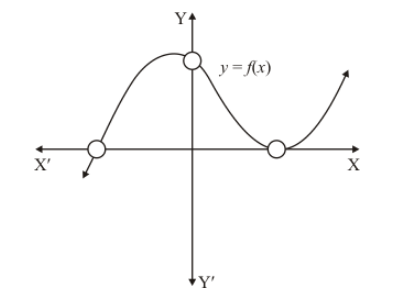# The graph of a polynomial y = f(x), shown in Fig. 2.18.`
Question:

The graph of a polynomial y = f(x), shown in Fig. 2.18. Find the number of real zeros of f(x).Solution:

A real number $\alpha$ is a zero of polynomial $f(x)$, if $f(\alpha)=0$In the above figure the curve intersects x-axis at one point and touches at one point

When a curve touches x-axis at one point, it means it has two common zeros at that point

Hence the number of real zeroes is 3